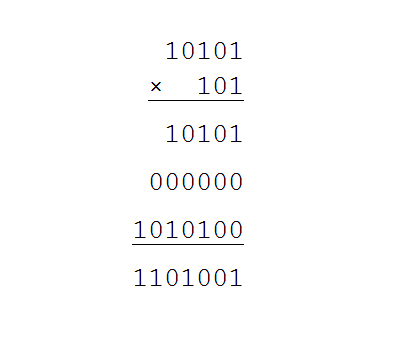Education ∪ Math ∪ Technology

A binary number is a number written in base 2 format, like 101010101111.  The binary number system is handy because it can be easily related to logical operators used in circuitry, and so almost all modern computers use this format for communication.

We use the decimal system for communication in our day to day lives because it is related to our original numbering systems, and this entire system was developed because of we have ten fingers between our two hands.

To convert a decimal number to a binary number, we want to rewrite the decimal number as a sum of powers of 2. For example, the number 5 is equal to 4 + 1 or 22 + 20 which is the same as 1×22 + 0×21 + 1×20. In binary, we write 5 as 101, since those are the coefficients of the powers of 2 (Try out this application which lets you switch between binary and decimal numbers).

Here is the basic multiplication table for binary, which only includes 0 and 1, since those are the only digits you have to multiply in binary (in a decimal system, you need a much larger multiplication table, since you need to be able to multiply each of 10 different digits, 0 – 9 by each of 10 different digits).

 × 0 1 0 0 0 1 0 1

Compare this to the traditional 10 by 10 multiplication table for decimal numbers.(Image credit: valilouve)

If you want to multiply numbers in binary, you could use some similar strategies to regular decimal multiplication. For example, 10101 times 101 looks like this:If you want to double check, 10101 is the same as 1×24 + 0×23 + 1×22 + 0×21 + 1×20 = 16 + 0 + 4 + 0 + 1 = 21 and 101 is 5 (as we noted before) so this multiplication in decimal is 21×5, which is 105. 1101001 = 1×26 + 1×25 + 0×24 + 1×23 + 0×22 + 0×21 + 1×20 = 64 + 32 + 8 + 1 = 105. See this website for a more detailed example of binary multiplication.

The point of this activity is that you have taken something which is hard to do (memorizing a 10 by 10 times table) and switched it to something which is conceptually more difficult, but easier to memorize. For smaller numbers, it is faster to multiply directly in decimal, but for larger numbers, it will actually take less time to convert them to binary, do the multiplication, and convert back. You may notice that the multiplication step itself is much easier than decimal multiplication, since it’s just a matter of remembering 2 facts (0×0 = 0 and 0×1 = 1) and lining up the numbers correctly so that the place value matches. Check this page out for more information on binary number operations.

If all of this feels arbitrary and bizarre to you, now you know what many 3rd graders feel like when they are first introduced to multiplication.